## How to Calculate and Solve for Reaction: Lift Falls Freely | Motion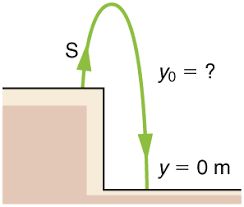The image above represents reaction: lift falls freely.

To compute for reaction: lift falls freely, two essential parameters are needed and these parameters are mass (m) and acceleration due to gravity (g).

The formula for calculating reaction: lift falls freely:

R = mg

Where;

R = Reaction
m = Mass
g = acceleration due to gravity

Let’s solve an example;
Find the reaction when the mass is 30 and acceleration due to gravity is 9.8.

This implies that;

m = Mass = 30
g = acceleration due to gravity = 9.8

R = mg
R = 30 x 9.8
R = 294

Therefore, the reaction is 294 N.

Calculating the Mass when the Reaction and the Acceleration due to Gravity is Given.

m = R / g

Where;

m = Mass
R = Reaction
g = acceleration due to gravity

Let’s solve an example;
Find the mass when the reaction is 40 and the acceleration due to gravity is 9.8

This implies that;

R = Reaction = 40
g = acceleration due to gravity = 9.8

m = R / g
m = 40 / 9.8
m = 40 / 9.8
m = 4.08

Therefore, the mass is 4.08.

## How to Calculate and Solve for Reaction: Lift Moves Down | Motion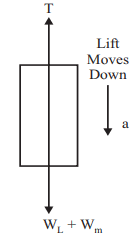The image above represents reaction: lift moves down.

To compute for reaction: lift moves down, three essential parameters are needed and these parameters are mass (m), acceleration (a) and acceleration due to gravity (g).

The formula for calculating reaction: lift moves down:

R = m(g – a)

Where;

R = Reaction
m = Mass
g = acceleration due to gravity
a = Acceleration

Let’s solve an example;
Find the reaction when the mass is 28, acceleration is 9 and acceleration due to gravity is 9.8.

This implies that;

m = Mass = 28
g = acceleration due to gravity = 9.8
a = Acceleration = 9

R = m(g – a)
R = 28(9.8 – 9)
R = 28(0.80)
R = 22.4

Therefore, the reaction is 22.4 N.

Calculating the Mass when the Reaction, the Acceleration and the Acceleration due to Gravity is Given.

m = R / g – a

Where;

m = Mass
R = Reaction
g = acceleration due to gravity
a = Acceleration

Let’s solve an example;
Find the mass when the reaction is 42, the acceleration is 8 and the acceleration due to gravity is 9.8

This implies that;

R = Reaction = 42
g = acceleration due to gravity = 9.8
a = Acceleration = 8

m = R / g – a
m = 42 / 9.8 + 8
m = 42 / 17.8
m = 2.359

Therefore, the mass is 2.359.

## How to Calculate and Solve for Reaction: Lift Moves Up | Motion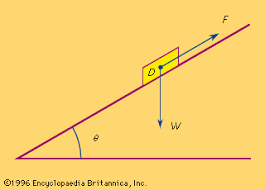The image above represents reaction: lift moves up.

To compute for reaction: lift moves up, three essential parameters are needed and these parameters are mass (m), acceleration (a) and acceleration due to gravity (g).

The formula for calculating reaction: lift moves up:

R = m(a + g)

Where;

R = Reaction
m = Mass
g = acceleration due to gravity
a = Acceleration

Let’s solve an example;
Find the reaction when the mass is 18, acceleration is 21 and acceleration due to gravity is 9.8.

This implies that;

m = Mass = 18
g = acceleration due to gravity = 21
a = Acceleration = 9.8

R = m(a + g)
R = 18(21 + 9.8)
R = 18(30.8)
R = 554.4

Therefore, the reaction is 554.4 N.

Calculating the Mass when the Reaction, the Acceleration and the Acceleration due to Gravity is Given.

m = R / a + g

Where;

m = Mass
R = Reaction
g = acceleration due to gravity
a = Acceleration

Let’s solve an example;
Find the mass when the reaction is 42, the acceleration is 21 and the acceleration due to gravity is 9.8

This implies that;

R = Reaction = 42
g = acceleration due to gravity = 9.8
a = Acceleration = 21

m = R / a + g
m = 42 / 21 + 9.8
m = 42 / 30.8
m = 1.36

Therefore, the mass is 1.36.

## How to Calculate and Solve for Recoil Velocity | Motion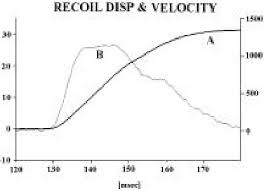The image above represents recoil velocity.

To compute for recoil velocity, three essential parameters are needed and these parameters are M, V and m.

The formula for calculating recoil velocity:

v = (-(M) x V) / m

Let’s solve an example;
Find the recoil velocity when M is 24, V is 10 and m is 6.

This implies that;

M = 24
V = 10
m = 6

v = (-(24) x 10) / 6
v = (-240) / 6
v = -40

Therefore, the recoil velocity is -40.

## How to Calculate and Solve for Force | Motion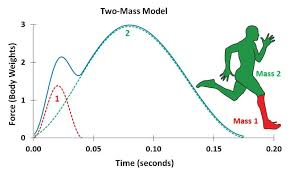The image above represents force.

To compute for force, two essential parameters are needed and these parameters are mass (m) and acceleration (a).

The formula for calculating force:

F = ma

Where;

F = Force
m = Mass
a = Acceleration

Let’s solve an example;
Find the force when the mass is 12 and the acceleration is 26?

This implies that;

m = Mass = 12
a = Acceleration = 26

F = ma
F = 12 x 26
F = 312

Therefore, the force is 312 N.

Calculating for Mass when the Force and the Acceleration is Given.

m = F / a

Where;

m = Mass
F = Force
a = Acceleration

Let’s solve an example;
Find the mass when the force is 100 and the acceleration is 20.

This implies that;

F = Force = 100
a = Acceleration = 20

m = F / a
m = 100 / 20
m = 5

Therefore, the mass is 5.